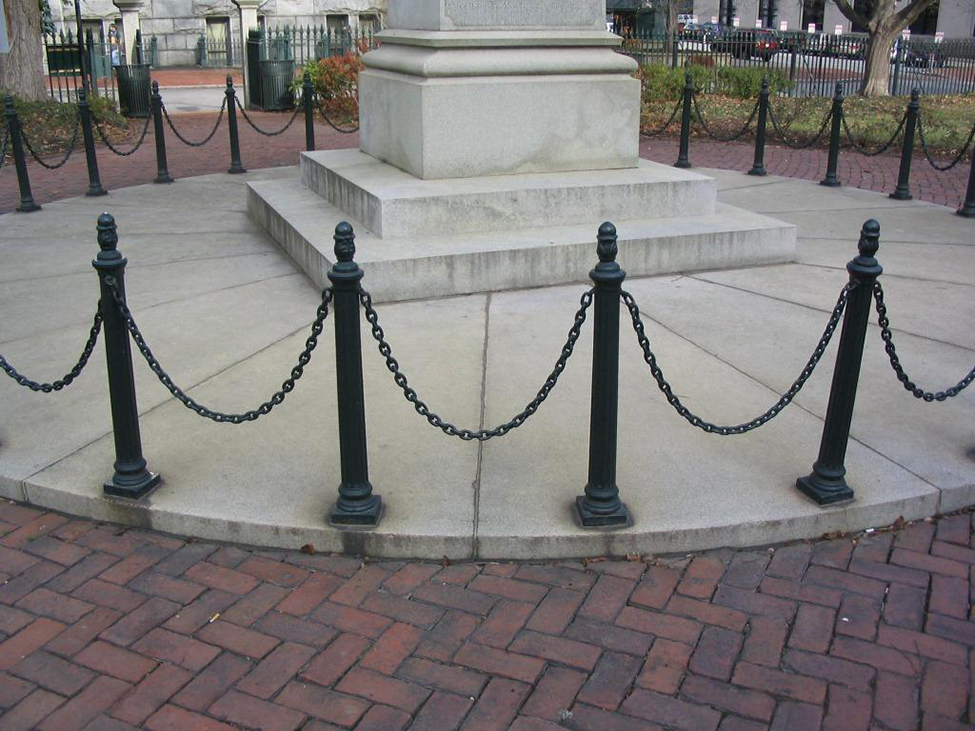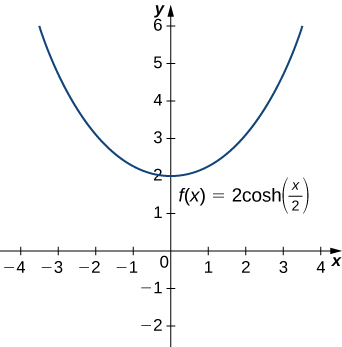## Applications of Hyperbolic Functions

### Learning Outcomes

• Describe the common applied conditions of a catenary curve

One physical application of hyperbolic functions involves hanging cables. If a cable of uniform density is suspended between two supports without any load other than its own weight, the cable forms a curve called a catenary. High-voltage power lines, chains hanging between two posts, and strands of a spider’s web all form catenaries. The following figure shows chains hanging from a row of posts.Figure 3. Chains between these posts take the shape of a catenary. (credit: modification of work by OKFoundryCompany, Flickr)

Hyperbolic functions can be used to model catenaries. Specifically, functions of the form $y=a\text{cosh}\left(\frac{x}{a}\right)$ are catenaries. Figure 4 shows the graph of $y=2\text{cosh}\left(\frac{x}{2}\right).$Figure 4. A hyperbolic cosine function forms the shape of a catenary.

### example: Using a Catenary to Find the Length of a Cable

Assume a hanging cable has the shape $10\text{cosh}\left(\frac{x}{10}\right)$ for $-15\le x\le 15,$ where $x$ is measured in feet. Determine the length of the cable (in feet).

Watch the following video to see the worked solution to Example: Using a Catenary to Find the Length of a Cable.

### Try It

Assume a hanging cable has the shape $15\text{cosh}\left(\frac{x}{15}\right)$ for $-20\le x\le 20.$ Determine the length of the cable (in feet).